BAKI DÖVLƏT UNİVERSİTETİ
TƏTBİQİ RİYAZİYYAT
ELMİ TƏDQİQAT İNSTİTUTU

## Seminars

The weekly scientific seminar devoted to the modern problems of applied mathematics is held.
on Tuesday, at 1000 o'clock.

Interested persons can take part

13.09.2022 the Institute will hold a webinar via video conference

Lector: Guljigit  Toktoshov

Theme: An approach for the topology design and optimization of a utility network with unreliable elements

Abstract: We consider a problem of the topology design and optimization of a utility communication network. Mathematically, it is represented by a hierarchical two-level network which allows us to take into account the fact that a communication line (trench or tunnel) of a primary network can be used multiple times for laying edges (various utility communications) of a secondary network while the expenses for preparation of that line are made just once. Another advantage is that we can consider a failure in a primary network along with its influence on all secondary network elements lying in it. For a given topology of a secondary network and a redundant topology of a primary network, we analyze the complexity of the problem of the cheapest choice of the primary network elements for laying into them secondary network elements. The methods for solving this problem are proposed, along with results of the numerical experiments.

PDF fayl

__________________________

15.03.2022 the Institute will hold a webinar via video conference

Lector: Ali Shokri

Theme: P-stability in explicit linear multistep methods

Abstract: According to Lambert and Watson, says that the linear multistep P-stable methods can not be explicit; they must be implicit and being implicit is the essential condition to obtain important feature of P-stability. Few explicit P-stable methods have been created in which they are nonlinear or at most P-stable. For the first time in literature, in this paper, we create a new family of explicit linear two-step singularly P-stable methods with phase-lag of order infinity for the numerical solution of initial value problems of second-order ordinary differential equations. Finally, the numerical results obtained by the new family for some well-known problems show its superiority in efficiency, accuracy, convergency and stability.

______________________________________

22.02.2022 the Institute will hold a webinar via video conference

Lector: Vagif Guliyev

Theme: Generalized Sobolev–Morrey estimates for hypoelliptic operators on homogeneous groups

Generalized Sobolev–Morrey estimates for hypoelliptic operators on homogeneous groups

__________________________

08.02.2022 the Institute will hold a webinar via video conference

Lector: Reyhan Shikhlinskaya

Theme: The introduction of a fuzzy parameter to predict the devaluation of the local currency caused by the fall in world oil prices
Abstract: In the presented article, the forecast of the USD / AZN currency quotation is considered using fuzzy time series (FTS). The novelty of this study in the introduction of the new parameter, to reflect the devaluations of 2015 and 2016, resulting from a sharp drop in oil prices. In particular, it takes into account the fact that Azerbaijani manat is a commodity currency, and the against the dollar to manat depends on the cost of oil and is regulated by the state.
_________________________

25.01.2022 the Institute will hold a webinar via video conference

Lector: Rafael Hamidov

Theme: One dynamic linear parametric programming and its solution

Abstract: A large-scale parametric programming problem is considered. The problem has a practical content. We develop the procedure for its solution. The proposed procedure first reduces initial problem to smaller-scale problems. With the help of their solutions we set the Pareto boundary of the two criteria problem, established against the initial problem. Then, with the help of this boundary the initial problem is solved.

_______________________

18.01.2022 the Institute will hold a webinar via video conference

Lector: Nihan Aliyev

Theme: From archimedes to powerative integral

Abstract: The paper is devoted to one direction of modern mathematics taking its origin from additive analysis, later from multiplicative analysis and, finally, powerative analysis. For each case there is given a direct operation, an integral and the inverse operative.

__________________________________

28.12.2021 the Institute will hold a webinar via video conference

Lector: Shargiyya Faracova

Theme: Discussion of the dissertation on the topic of Solving of stabilization problems with output variable the frequency and time calculation methods

Abstract: The work consists of the development of algorithms for solving time stabilization problems, time and frequency calculation methods, algorithms for solving the problem of three-point boundary conditional optimization, which is not separated at the internal and end points, and the application of the results to gas extraction.

___________________________

21.12.2021 the Institute will hold a webinar via video conference

Lector: Hajiyeva Nazıle

Theme: Determınatıon the coeffıcıent of hydraulıc resıstance ın two dıfferent parts of the well ın dıscrete case of gas lıft process

Abstract: In the paper the time-averaged mathematical model of the gas-lift process is considered. At the first iteration, using a small parameter (the small parameter is taken as the reciprocal of the well depth) the analytical expression for the gas-liquid mixture at the end of the lift is constructed and it is assumed that the coefficient of hydraulic resistance have different values in two different parts of the lift. The quadratic functional is constructed by means of the least squares method. Minimization of this functional with respect to the coefficient of hydraulic resistance gives us the desired result. On the basis of statistical data, asymptotic formulas for the coefficient of hydraulic resistance in two different parts of the lift are given. The results are illustrated with an example. It is shown that the obtained results coincide with the known results with an accuracy of 10-1.

__________________________________

14.12.2021 the Institute will hold a webinar via video conference

Lector: Ramazanov  Ali

Theme: About the steepness of the function of discrete argument

Abstract: We introduce the notion of steepness of a coordinate-convex function of discrete argument on an ordinal-convex set. In terms of guaranteed estimates it is shown that in problems of optimization of coordinate-convex functions on an ordinal-convex set the gradient coordinatewise lifting algorithm is stable under small disturbances of the steepness of the utility function.

____________________________

07.12.2021 the Institute will hold a webinar via video conference

Theme: Classic models of rationality, satisficing principle and sequential choice

Abstract: We discuss the variety of classic models of rationality, their different generalizations, the model of choice based on Simon’s Satisficing Principle, its connection with some versions of classic rationality. The model of superposition of choice functions is discussed, its rationality and efficiency. Several real-life examples illustrate the models.

________________________

23.11.2021 the Institute will hold a webinar via video conference

Lector: Mutallim Mutallimov

Theme: Development of algorıthms to control the spatıal motıon of the quadrocopter

Abstract: The recent development of unmanned aerial vehicles, including quadrocopters, makes it possible to use the latter in various fields of human activity. Lightness, small size, maneuverability, ease of control are the main advantages of quadcopters, which allow them to be used in many industries, including in the military field. The development of control algorithms for the dynamics of a quadcopter flight is carried out on the basis of an adequate mathematical model. As a numerical example, the control of the spatial motion of a quadrocopter is considered when working out a given trajectory.

_________________________

16.11.2021 the Institute will hold a webinar via video conference

Lector: Soltanova Vusala

Theme: Cauchy problem for two- dimensional second- order differential equation with discrete multiplicative and powerative derivatives.

Abstract: Presented work is devoted to the study of the solution of Cauchy problem for two- dimensional second- order differential equation with discrete multiplicative and discrete powerative derivatives on diffrent variables. General solution of the equation in question was determined, which contains some arbitrary constants. These arbitrary constants are determined using given initial data.

____________________

02.11.2021 the Institute will hold a webinar via video conference

Lector: Afanasyev Aleksandr Petrovic

Theme: Methods of multidimensional visualization for analysis and performance management of convex and non-convex production units

Abstract: Mathematical methods and models have been used for a long time for efficiency analysis and managerial performance of complex socio-economic units. For example, ratio analysis is widely used for efficiency analysis of such production units as financial institutions, regions, hospitals, universities and so on. In this approach, a set of ratios is introduced for every production unit. After this, all these ratios are added with some weights thus rating function is determined for evaluating the performance of every unit. Such an approach based on rating function is widely used so far in business informatics. In this presentation we are trying to show the development of methods of multidimensional visualization for analysis and performance management of convex and non-convex production units.

_________________________________________

19.10.2021 the Institute will hold a webinar via video conference

Theme: Reverse  displacement analisis of 6r serial manipulator by using dual quaternions

Abstract: The inverse problem of movements of a manipulator 6R of a sequential structure is considered. Double quaternions are used to derive algebraic equations. These equations were greatly simplified by introducing intermediate angles. Unknown are sines and cosines of intermediate angles, which are linear combinations of rotation angles in kinematic pairs. The first six of the 16 equations are linear with respect to the unknowns, the next 8 equations express the equality to unity of the sum of the squares of the sines and cosines, and the remaining 2 equations express additional connections between the unknowns. Numerical results are available.
_____________________________________

19.10.2021 the Institute will hold a webinar via video conference

Lector: Yagub Sharifov

Theme: Existence and uniqueness of the solutions of the nonlinear impulse differential equations with nonlocal boundary conditions

Abstract: In this paper the system of ordinary impulse differential equations with nonlocal conditions is investigated. First, the boundary value problem is reduced to the equivalent integral equation. Further, using the fixed point theorem, conditions for the existence and uniqueness of the solution of the boundary value problem are obtained. The continuous dependence of the solutions on the right-hand side of the boundary conditions is also established.

Existence and uniqueness of the solutions of the nonlinear impulse differential equations with nonlocal boundary conditions

___________________________

28.09.2021 the Institute will hold a webinar via video conference.

Theme: Development of a mathematical model of a differential piezoelectric converter and its computer simulation

Abstract: The urgency of the problem is justified and the problem statement is formulated. Using mathematical methods of measuring technology and the theory of piezoelectricity, a mathematical model of an electric energy converter is compiled taking into account the measured value - the effects of mechanical influences. The resulting mathematical model, the distinctive feature of which is that it includes only the electrophysical and geometric parameters of piezoelectric sensing elements with a differential connection, is considered as a functional dependence between the output electrical signal and the input relative signal of mechanical action. Based on the results of computer simulation of the compiled model, the necessary conclusions are drawn and a conclusion is formulated.

____________________________________

21.09.2021 the Institute will hold a webinar via video conference.

Lector: Fikter  Aliev

Theme: Some mathematical problems and their solutions for the oscillating systems with liquid dampers  (survey)

Abstract: The mathematical problem of an oscillating system with liquid dampers is considered, such as finding the order of the fractional derivative of a subordinate term based on the given statistical data from practice, constructing a solution of the corresponding system with nonseparated boundary conditions, including for large values of the head mass, finding asymptotic solutions on the first approximations, and constructing optimal controllers to stabilize the system  around the corresponding program trajectories and controls.

_____________________________

13.07.2021 the Institute will hold a webinar via video conference.

Lector: Aslanova  Nigar

Theme: On selfadjoint extension of symmetric operator with exit to larger space

Abstract: We investigate minimal operator corresponding to operator differential expression with , exit to larger space, study its selfadjoint extension, corresponding to boundary value problem with some rational function of eigenparameter in boundary condition, establish asymptotics of spectrum and derive trace formula.

_____________________________

06.07.2021 the Institute will hold a webinar via video conference.

Lector: Hajar  Movsumova

Theme: The asymptotic  of the eigenvalues for the bessel operator equation with an unbounded operator coefficient.

Abstract: The main goal  of  this article is to  investigate the boundary value problem with an eigenvalue dependent boundary condition for the Bessel operator equation with an unbounded operator coefficient. At first we  proved some spectral properties of  this problem. Also, the asymptotic behavier of the boundary value problem   investigated.

___________________________

01.06.2021 the Institute will hold a webinar via video conference.

Lector: Sabir Hamidov

Theme: Characteristic prices and growth rates In one Neumann type model

Abstract: Neumann type model of economic dynamics with n technologies is considered. Characteristic prices and growth rates of national wealth are determined with some conditions on trajectory. Superdifferentials of the maximum function of characteristic prices are found. Theorem on growth rate stability is proved.

Characteristic prices and growth rates In one Neumann type model

_____________________________

25.05.2021 the Institute will hold a webinar via video conference.

Theme: Ranking DMUS by Bootstrapping method

Abstract: The efficiency of Groups is determined by a comparison of their inputs and outputs. For multiple inputs and outputs such a comparison is not straightforward but can be accomplished using Data Envelopment Analysis. This is a technique based on linear programming which compares each unit with all the others and determines its efficiency in terms of other Groups with comparable inputs and outputs. This article suggests bootstrapping method for ranking measures of technical efficiency as calculated via Data Envelopment Analysis (DEA).  This technique is employed to evaluate the efficiency of 9 sections of hospitals in Ardabil.

RANKING DMUS BY BOOTSTRAPPING METHOD

__________________________________

18.05.2021 the Institute will hold a webinar via video conference.

Lector: Sh. Mustafayev

Theme: Ground equipment for collecting annular gas in wells

Abstract: There are a lot of wells with free gas in the content of product got from the layer among the rod borehole pump installations of  “Azneft” PU. But according to some  reasons, the necessary communications in the mines either are in the unsuitable conditions, or do not exist. That is why the backside of most wells operates in an open state that causes the environmental pollution and loss of valuable products. In order to prevent this, the pipe front space is often shut nermetically. However, this process is possible when the gas production  is less  (up  to 10, the extension causes the distortion of operating mode of the well.
​I reorder to liquidate the above mentioned shortcomings, the ground equipment has been developed. This equipment creates ad favorable operating mode in the well and at the same time prevents the loss of pipe front gas. This, it regulates the pipe front gas and provides the transmission of this gas to the discharge line and its transportation with production well.
​After the trimmed equipment was set in the 3304th well of “Bibiheybatneft” oil and Gas Production, it became possible not only to let gas into the air, but also to increase the oil production, and to decrease the number of underground constructions.

GROUND EQUIPMENT FOR COLLECTING ANNULAR GAS IN WELLS

___________________________________

20.04.2021 the Institute will hold a webinar via video conference.

Lector:  professor Arazov Hasanbay

Theme: Time in mathematical simulations of the theory of population of observed families of small bodies in non-linear dynamic systems

Abstract: Time continuously controls the variability of the observed natural resources; time teaches by numerous observable examples; time controls the movements of numerous objects at different speeds. Time discards unnecessary stresses in families of small bodies in dynamic systems. It plays the role of a cleaner from excess energies in nature. It leaves only really necessary tensions. Time always starts on time and stops in time. The beginning of time coincides with the beginning of the birth of the Universe. The end of time coincides with the end of the Universe's lifetime. The observed centuries-old systems of birth and evolution of compounds of elements of both living and non-living forms of matter over a millennium, in the dynamic systems of the family of populations of small bodies are indicated as example

TIME IN MATHEMATICAL SIMULATIONS OF THE THEORY OF POPULATION OF OBSERVED FAMILIES OF SMALL BODIES IN NON-LINEAR DYNAMIC SYSTEMS

_______________________________

13.04.2021 the Institute will hold a webinar via video conference.

Lector:  professor Gamzaev Kh.M.

Theme: Turbulent flow of fluid in the pipeline numerical modeling method

Abstract: In the report, the issues of numerical investigation of turbulent flow of bircins fluid in pipelines are considered. Using the theory of semi-empirical turbulence of prandatlin, a mathematical model of the flow is proposed, on the basis of which the question of determining the hydraulic characteristics of the pipeline is put. The numerical solution of this problem, which belongs to the class of inverse problems, is proposed. The report interprets the results of the computational experiment conducted on the model issue.

________________________

06.04.2021 the Institute will hold a webinar via video conference.

Lector:  professor Mahammad Camalbayov

Theme: NEW CONCEPT FOR IMITATION MODELING OF THE DYNAMIC SYSTEMS: Theory and Application

Abstract: A new simulation concept of dynamic systems is being developed. The main idea, terms of the concept and principles of creating a model of a complex physical process are presented, which imply the division of the process under consideration into separate objects connected with each other by a cause-and-effect relationship. The proposed concept is applied to modeling the process of developing a volatile oil reservoir operated by a well equipped with a submersible sucker rod pump in a pump-well-reservoir system. Algorithms have been developed to predict the main indicators of the process, taking into account the PVT properties of fluids and phase transformations of the hydrocarbon system during filtration in the reservoir. The presence of the annular space in the well and the crossflow between the annular space and the lift pipe are taken into account. A number of computer studies were carried out on the basis of the developed model.

________________________________

16.03.2021 the Institute will hold a webinar via video conference.

Lector:  Dr. Maryam Molayi

Theme: The new class of multistep multiderivative hybrid methods for the numerical solution of chemical stiff systems of first order IVPs.

Abstract: In this paper, we present a general form of Nth derivative multistep methods. In these hybrid multistep multiderivative methods, additional stage points (or off-step points) have been used in the first derivative of the solution to improve the absolute stability regions. The accuracy and stability properties of these methods are investigated. We apply the new methods for the numerical integration of some famous stiff chemical problems such as Belousov–Zhabotinskii reaction, the Chapman atmosphere, chemical Akzo-Nobel problem, ROBER problem (suggested by Robertson) and some others which are widely used in numerical studies.

__________________________________________

02.03.2021  the Institute will hold a webinar via video conference.

Lector: Dr. Reza Şokri

Theme: Efficient explicit nonstandard finite difference scheme with positivity-preserving  propert.

Abstract: Solving problems in various fields of science and engineering, such as physical and biological systems, leads to solving parabolic equations of the advectiondiffusion reaction (ADR) type. In these problems, usually the concentration of chemical compounds or the size of the population are our unknowns, which are positive in nature. In general, the use of common methods, such as the classical finite difference method, may produce numerical drawbacks such as spurious oscillations and negative values in the solutions due to truncation errors. By using the nonstandard finite difference (NSFD) method, a better finite difference model is constructed. The proposed NSFD scheme ensures that the solutions are positive and there are no spurious oscillations in the solutions.

____________________________________

23.02.2021  the Institute will hold a webinar via video conference.

Lector: professoe Yagub Sharifov

Theme: Existence and uniqueness of the solution of nonlinear impulsive differential equations of the first order of multipoint boundary conditional

Abstract: The existence and uniqueness of the solution of the first-order solution of nonlinear impulsive differential equations with multiple point boundary conditions is investigated. The given initial boundary value problem is reduced to an integral equation equivalent to it. The existence and uniqueness of the solution is based on Banach's principle of compressed reflection. The existence of the solution is based on Krasnoselsky's and Schaufer's theorems on fixed points.

______________________

16.02.2021  the Institute will hold a webinar via video conference.

Lector: Ahmet Ocak Akdemir, Professor of Mathematics, Ataturk University, will deliver a speech entitled "New integral inequalities for Atangana-Baleanu fractional integral operators".

Theme: NEW INTEGRAL INEQUALITIES FOR ATANGANA-BALEANU FRACTIONAL INTEGRAL OPERATORS

Abstract. Integral identities created in inequality theory studies help to prove many inequalities. Recently, different fractional integral and derivative operators have been used to achieve these identities. In this article, with the help of Atangana-Baleanu integral operators, an integral identity was first obtained and various integral inequalities for convex functions have been proved   using this identity.

NEW INTEGRAL INEQUALITIES FOR ATANGANA-BALEANU FRACTIONAL INTEGRAL OPERATORS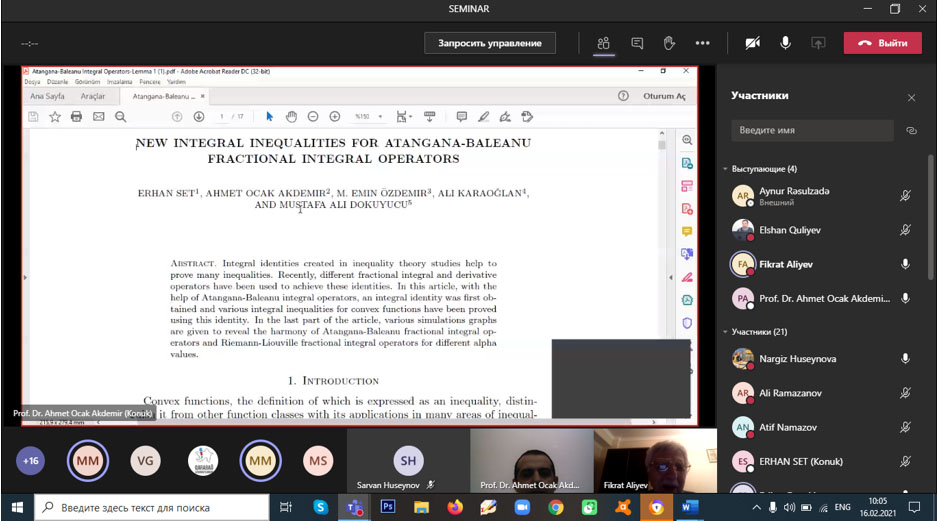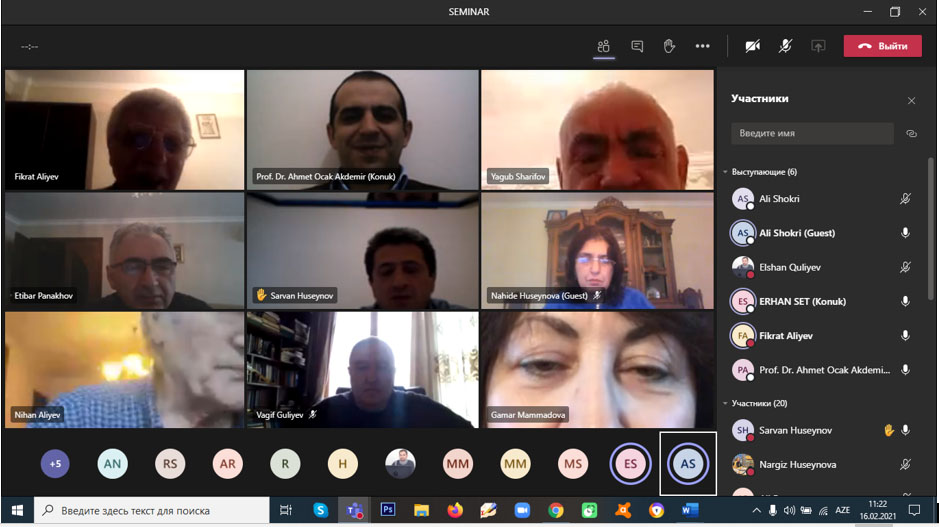________________________________

08.05.2018

Theme: An approach to numerical solution to inverse-coefficient problems for a parabolic equation

Lector: Leading scientist of The Institute of Control Systems of ANAS,  Anar Rahimov

The inverse problems are considered for a parabolic equation with an unknown source (space or time dependent) on the right-hand side. In particular, these classes of problems arise in the study of boundary value problems with nonlocal (integral) conditions. A numerical method is proposed to solve the problems, which is based on the use of the method of lines. The initial problems are reduced to a system of ordinary differential equations with unknown parameters. To determine unknown parameters non-iterative method is proposed. We present the results of numerical experiments on test problems.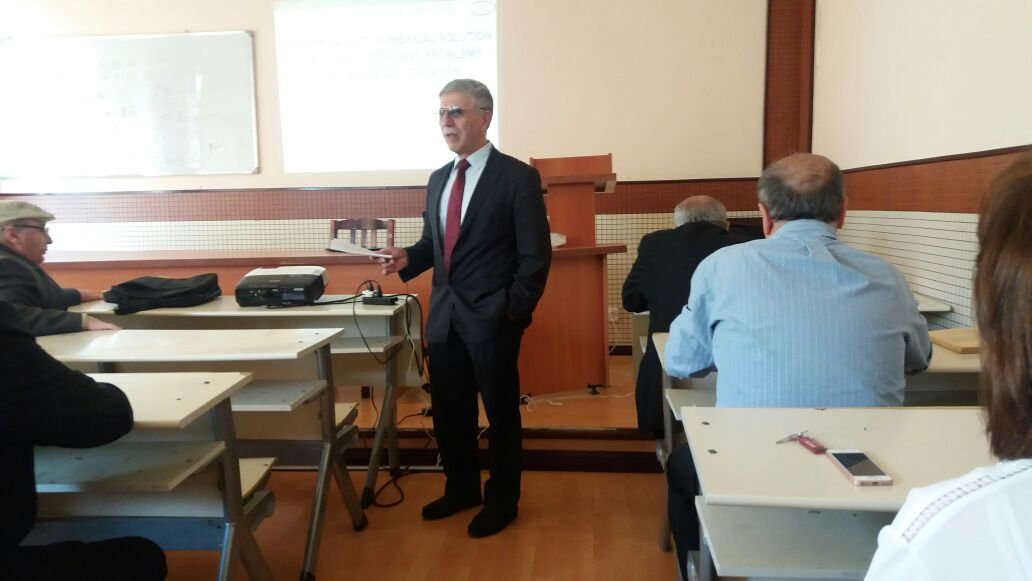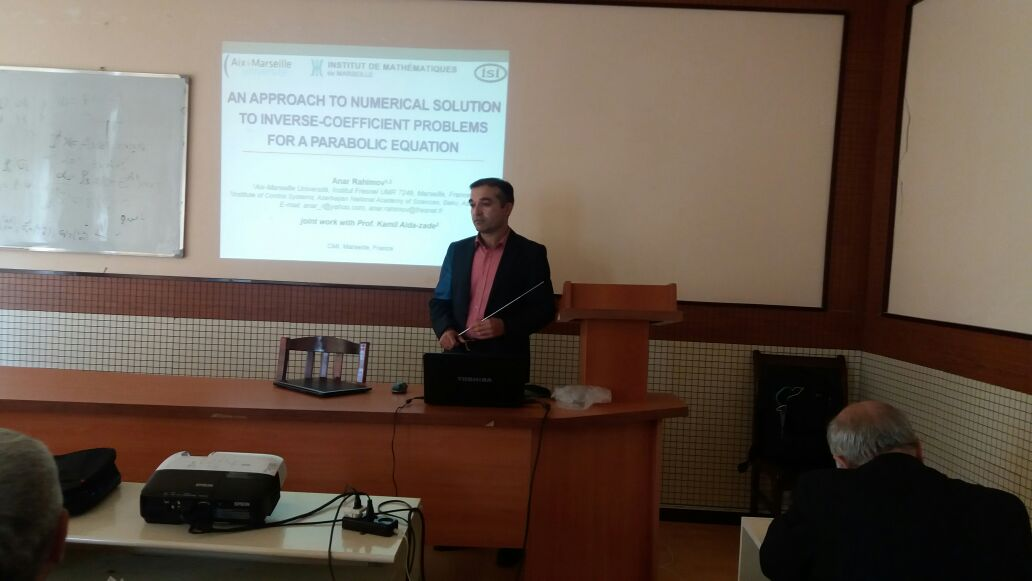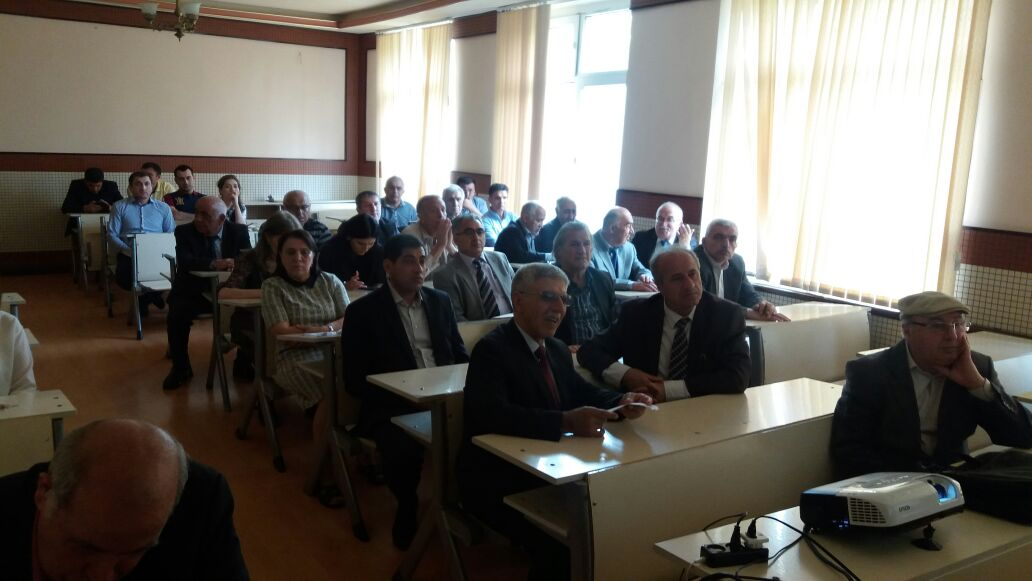__________________________

24.04.2018

Theme: Applying the theory of fuzzy time series in forecasting of the rate of USD / AZN

Lector: Shikhlinskaya R.Y.

In the presented article, forecasting of the currency quotation USD / AZN is considered using fuzzy time series (FTS). It provides a step by step description of the technique, which represents the construction of fuzzy term sets for the linguistic variable "exchange rate”, using input data as numerical values and calculating relationship to predict future states.  In particular, the fact that Azerbaijani manat is a commodity currency is taken into account and the dollar exchange rate for manat depends on the cost of oil and is regulated by the state. Forecasting FTS is carried out in the direction of the application of new methods, called Times-Series Data Mining.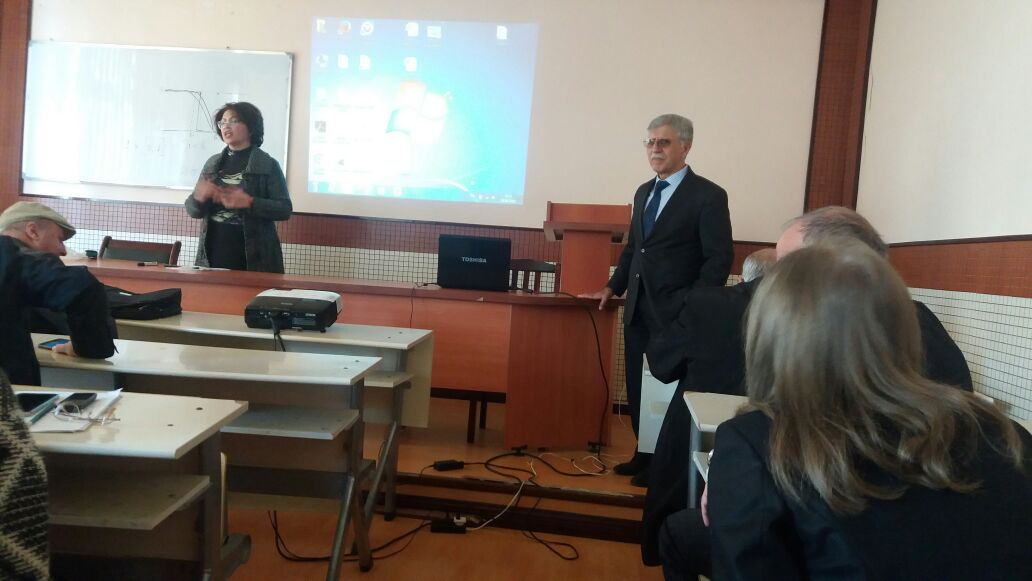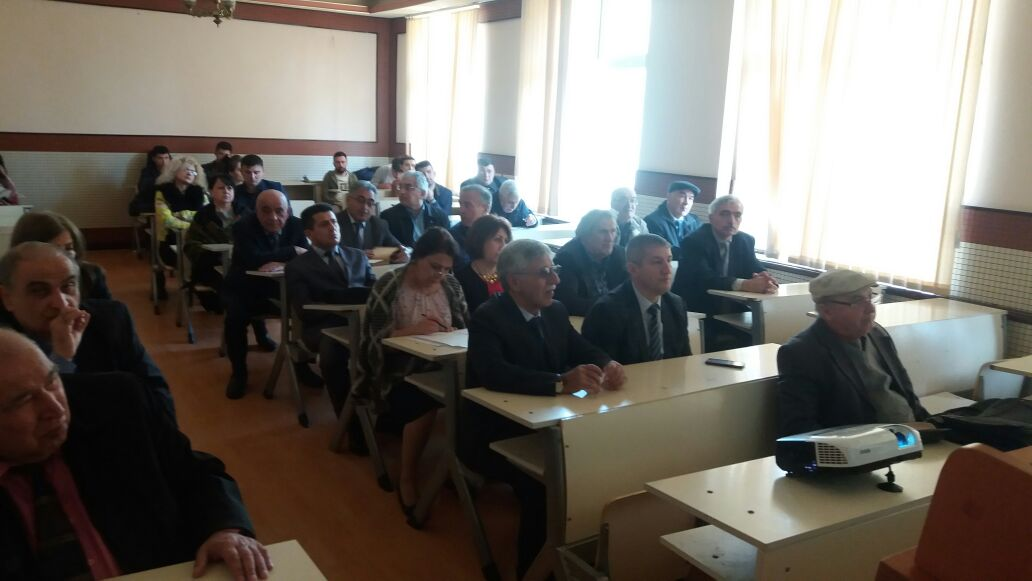__________________________

06.02.2018

Theme: Analytical construction of regulators for systems with fractional derivatives

Lector:  Fikret Aliev

The problem of analytic construction of regulators (AKP) is considered when the motion of an object is described by a system of linear differential equations of fractional derivatives with constant coefficients. Expressions are given for an analogous (ordinary derivative) Euler-Lagrange equation, where the fundamental matrix-solution is constructed using the Mittag-Leffler function. The above scheme is such that the order of the derivative. An example illustrating the proposed method is given.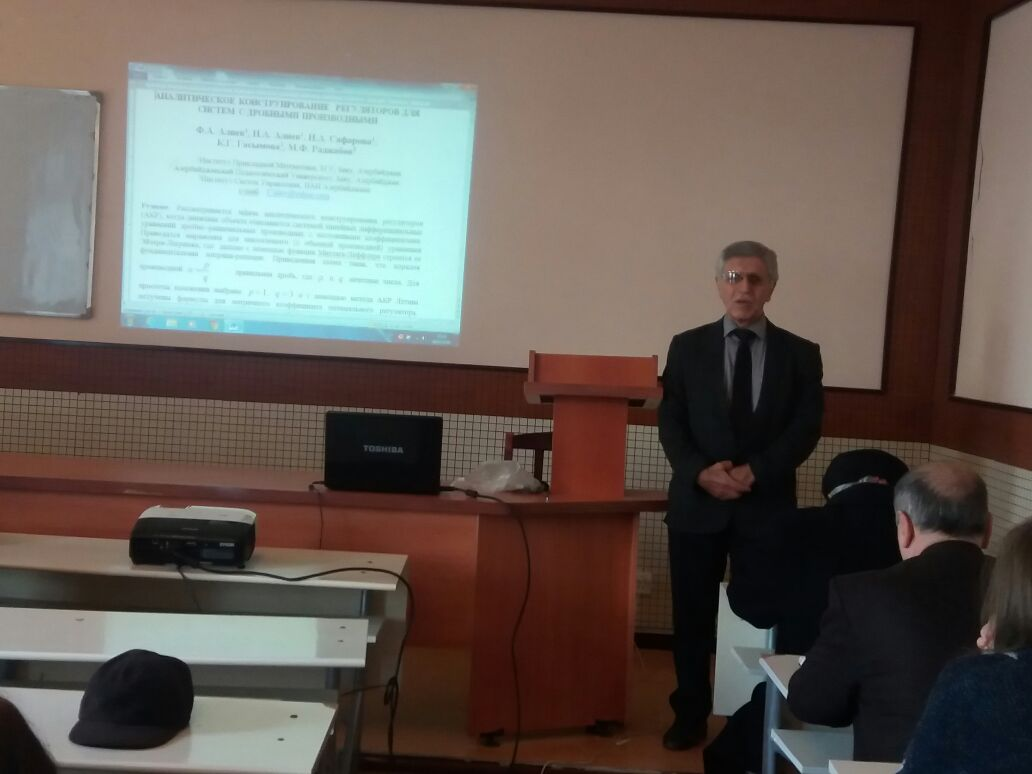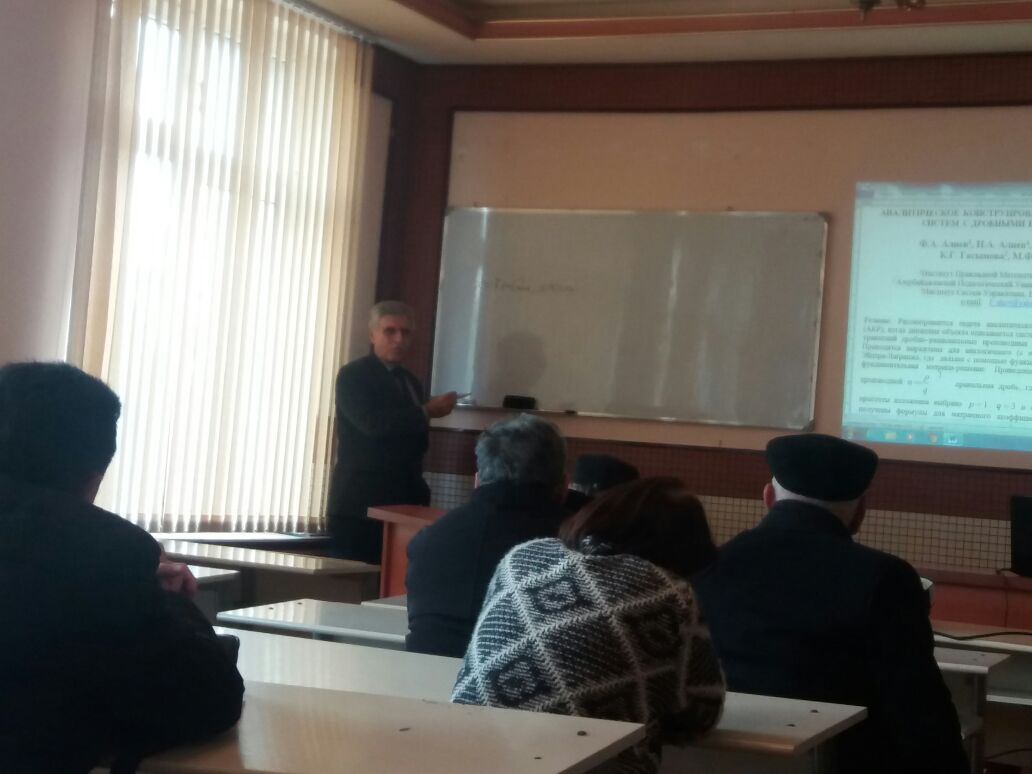_____________________

13.02.2018

Theme:  Identification problem for defining the coefficient of hydraulic resistance on different areas of pump-compressor pipes in gaslift process

Lector: I. Maharramov

In the work the coefficient of hydraulic resistance on different areas of pumpcompressor pipes in the oil production in gas lift wells is considered. By means of the least-squares method, the coefficient of hydraulic resistance is obtained using statistical data. An example is given that shows the adequacy of the mathematical model.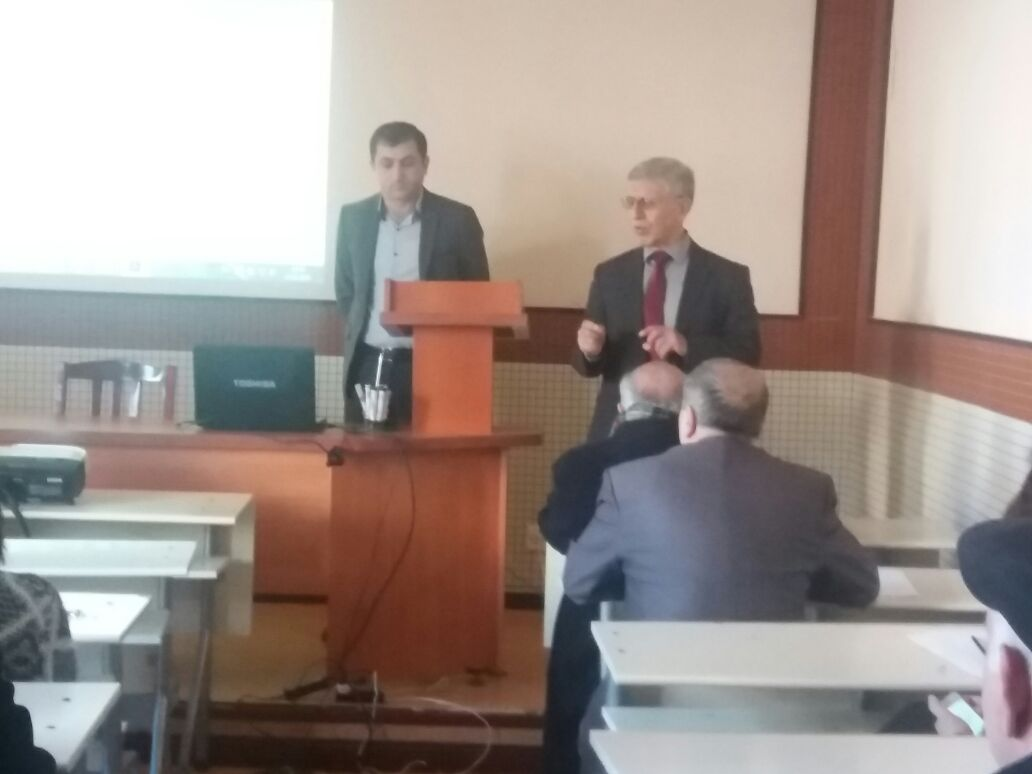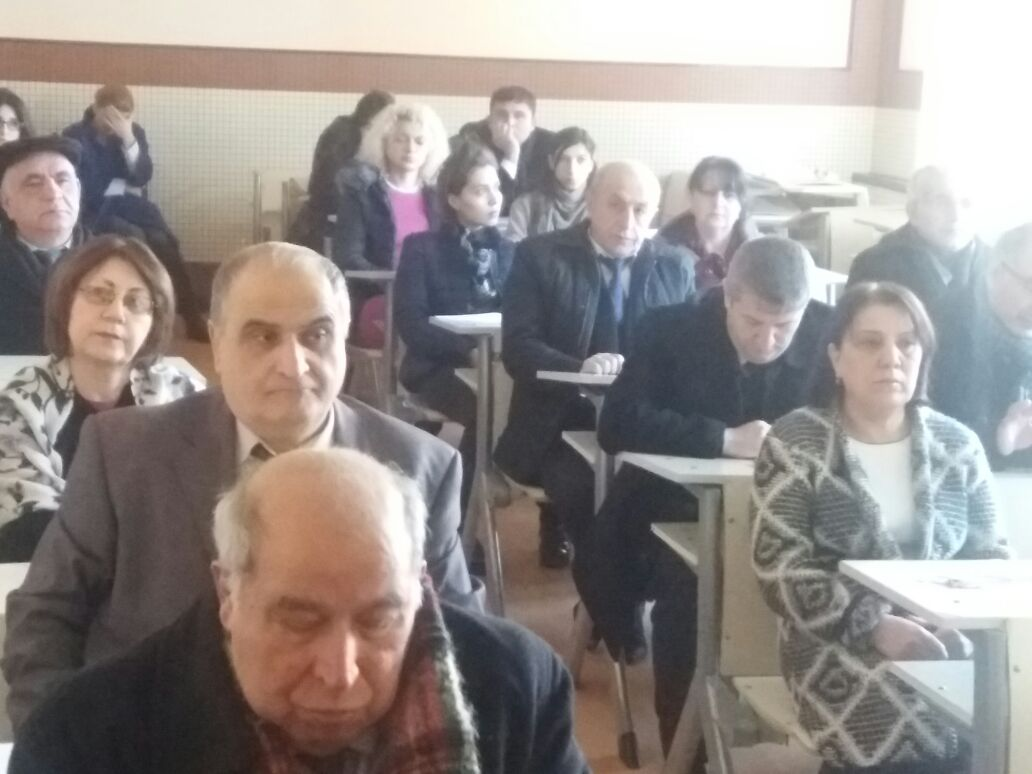Archive

The urgency of the problem is justified and the problem statement is formulated. Using mathematical methods of measuring technology and the theory of piezoelectricity, a mathematical model of an electric energy converter is compiled taking into account the measured value - the effects of mechanical influences. The resulting mathematical model, the distinctive feature of which is that it includes only the electrophysical and geometric parameters of piezoelectric sensing elements with a differential connection, is considered as a functional dependence between the output electrical signal and the input relative signal of mechanical action. Based on the results of computer simulation of the compiled model, the necessary conclusions are drawn and a conclusion is formulated.